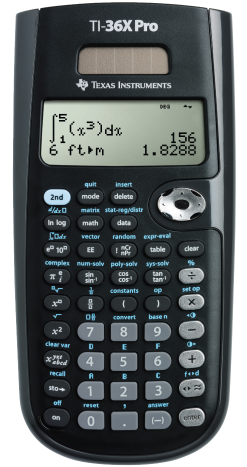Navigate: StoreFront / Hardware / Calculators / Texas Instruments TI-36X Pro Scientific Calculator
 < Previous Product Next Product >

# Texas Instruments TI-36X Pro Scientific Calculator

Price: \$24.95
 The TI-36X Pro Scientific Calculator is an advanced, four-line scientific calculator, with higher-level math and science functionality, is ideal for computer science and engineering courses in which graphing technology may not be permitted. The TI-36X Pro scientific calculator is approved for use on SAT®, ACT®, and AP® exams.Features include: View Multiple Calculations at One TimeCompare results and explore patterns on-screen with the MultiView™ display, which supports up to four lines. See Math Exactly as it Appears in Textbooks See math expressions, symbols and stacked fractions exactly the way they appear in textbooks — no need to adapt to a technical syntax; also provides quick access to frequently used functions. Built-In Solvers Intuitive prompts allow one to easily solve numeric equation, polynomial and system of linear equations. Easy-to-Use Mode Menu The menu is structured for easy, intuitive access to commands; select degrees/radians, floating/fix, Classic/MathPrint™ feature or number format. Explore (x,y) Tables of Values Students can easily explore an (x,y) table of values for a given function, automatically or by entering specific x values. Key Features: Four-line display One- and two-variable statistics MultiView™ display shows multiple calculations at the same time on screen Select degrees/radians, floating/fix, number format modes Choose from three solvers: numeric equation, polynomial and system of linear equations Display a defined function in a tabular form Determine the numeric derivative and integral for real functions Perform vectors and matrices using a vector and matrix entry window Ideal For: Algebra I and II Geometry Trigonometry Statistics Calculus Biology Chemistry Physics Computer science College math College science College engineering